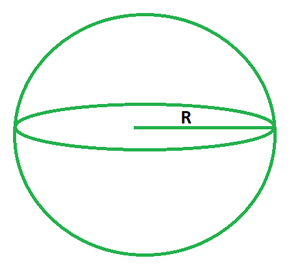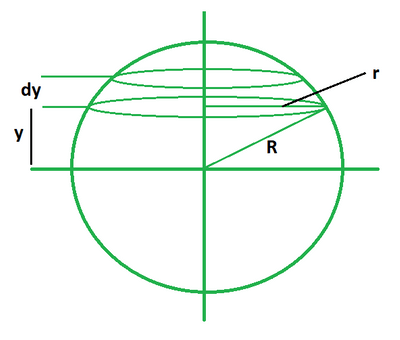# How to find the Volume of a Sphere?

• Last Updated : 30 May, 2022

A sphere is defined as a three-dimensional solid object such that all points on its surface are equidistant from its center. In other words, it is defined as a group of points in three-dimensional space that are all at the same distance from a given point, similar to a circle, which is geometrically a two-dimensional object. The distance between any point on a sphere’s surface and its center is called its radius.### Volume of a sphere formula

The volume of a sphere equals the amount of space occupied by it. It determines the area or region enclosed by the sphere. Its formula is equal to four times the product of pi and cube of radius divided by 3. Its standard unit of measurement is cubic meters (m3).

V = 4πR3/3

Where,

• V is the volume of sphere,
• π is a constant with the value of 3.14,
• R is the radius of sphere.

DerivationThe formula for volume of sphere is derived by using the method of integration. Consider a sphere of radius R divided into many small circular discs of radius r and thickness dy.

Volume of a circular disc, dV = πr2dy

=> dV = π (R2 – y2) dy

The total volume of the sphere can be calculated by adding the volume of all such possible discs. In other words, we have to integrate the above volume integral from lower limit -R to upper limit +R. So, the volume of sphere is calculated as,This derives the formula for volume of a sphere.

### How to Find the Volume of a Sphere?

Let’s take an example to understand how we can calculate the volume of a sphere.

Example: Calculate the volume of a sphere of radius 3 m.

• Step 1: Observe the radius of sphere. In this example, the radius of the sphere is 3 m.
• Step 2: It is known that the volume of a sphere is equal to 4πR3/3. Put the given value of radius in the formula.
• Step 3: So, the volume of sphere is calculated as, (4/3) × 3.14 × (3)3 = 113.09 cu. m

### Sample Problems

Problem 1: Calculate the volume of a sphere of radius of 5 m.

Solution:

R = 5

Using the formula we get,

V = 4πR3/3

= (4/3) × 3.14 × (5)3

= 523.59 cu. m

Problem 2: Calculate the volume of a sphere of radius 8 m.

Solution:

We have,

R = 8

Using the formula we get,

V = 4πR3/3

= (4/3) × 3.14 × (8)3

= 2144.66 cu. m

Problem 3: Calculate the volume of a sphere of radius 12 m.

Solution:

We have,

R = 12

Using the formula we get,

V = 4πR3/3

= (4/3) × 3.14 × (12)3

= 7238.22 cu. m

Problem 4: Calculate the volume of a sphere of radius 23 m.

Solution:

We have,

R = 23

Using the formula we get,

V = 4πR3/3

= (4/3) × 3.14 × (23)3

= 50965.01 cu. m

Problem 5: Calculate the radius of a sphere if its volume is 300 m3.

Solution:

We have,

V = 300

Using the formula we get,

V = 4πR3/3

=> R3 = 3V/4π

=> R3 = (3 × 300) / (4 × 3.14)

=> R = 4.15 m

Problem 6: Calculate the radius of a sphere if its volume is 670 m3.

Solution:

We have,

V = 670

Using the formula we get,

V = 4πR3/3

=> R3 = 3V/4π

=> R3 = (3 × 670) / (4 × 3.14)

=> R = 5.42 m

Problem 7: Calculate the diameter of a sphere if its volume is 1020 m3.

Solution:

We have,

V = 1020

Calculate the radius of the sphere.

V = 4πR3/3

=> R3 = 3V/4π

=> R3 = (3 × 1020) / (4 × 3.14)

=> R = 6.24 m

So, the diameter is calculated as,

d = 2r

= 2 (6.24)

= 12.48 m

My Personal Notes arrow_drop_up